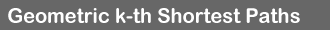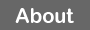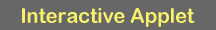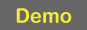What? This applet visualizes construction of the "k-th homotopic shortest path map" in a polygonal domain P with holes. Technical details about the applet are in this 2-pager. Formal details about the map can be found in this paper. Below is a brief self-contained explanation. Shortest path map All paths are assumed to start from a fixed point s in P (the green dot). Two paths to a point q are homotopically different (or have different homotopy types) if one cannot be continuously deformed to the other without intersecting holes. The 1st shortest path (or the 1-path) to q is just the shortest path from s to q. Say that q is on a 1-wall (depicted in red) if there exist two (necessarily homotopically different) 1-paths to it. The homotopic shortest path map of P (or 1-map) is P cut along 1-walls (the map is a simple polygon since there is a unique 1-path to every point in it).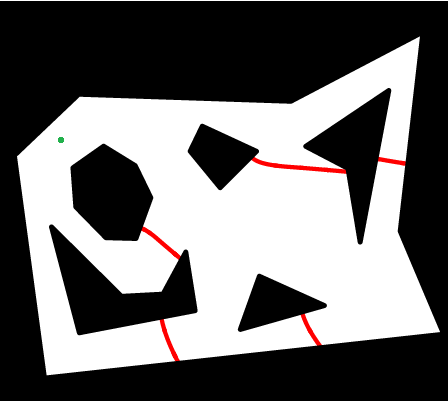k-paths The following notions are formally defined in this paper: The 2nd shortest path (or the 2-path) is the shortest path homotopically different from the 1-path. The k-th shortest path (the k-path) is defined recursively. The figure shows k-paths (p1, p2, p3, p4, p5) for k=1...5 (the 5-path is nonsimple -- it is equal to the 4-path plus the loop around the hole).Continuous Dijkstra Imagine that a wave starts propagating from s at unit speed. At any time t the wavefront consists of the points at geodesic distance t from s. The wavefront is composed from circular-arc wavelets. When wavelets collide their intersection point traces a 1-wall. The wavelets do not propagate over the walls; i.e., the part of any wavelet on the other side of the wall dies. The figure shows a snapshot of the propagation; the area claimed by the wave is gray.2-garage and wavelet parts resurrection Let's allow wavelets to propagate over the walls; the propagation, however, will continue not in the domain P but on the "next floor". Specifically, we define "parking garages" as follows: The 1-garage is just P. Recall that 1-map is P sliced along 1-walls. The 1-map will be the 1st floor (or 1-floor) of the 2-garage. Take a copy of the 1-floor and put it on top of itself; the copy will be the 2-floor -- the last floor of the 2-garage. The 1- and the 2-floors are glued along 1-walls; each side of any wall on the 1-floor is glued to the opposite side of the wall on the 2-floor. This way, when two wavelets meet at the 1-wall on the 1-floor, each continues propagating on the 2-floor (where the wavelets actually diverge since they propagate into different sides of the wall). When wavelets collide on the 2-floor, their meeting point traces a 2-wall. The next figure shows wavelets on the 2-floor in the instance from the previous figure (the shown parts of the wavelets would die if there were no 2-floor). The 1-walls are now blue, and the 2-walls are red.k-garage The k-garage for arbitrary k is defined recursively: Take the top floor (the (k-1)-floor) of the (k-1)-garage, slice it along (k-1)-walls, put a copy of the sliced floor on top of the (k-1)-garage, and glue the copy to the (k-1)-floor along (k-1)-walls, identifying the opposite sides of every wall. The copy is the k-floor -- the top floor of the k-garage. This way, when two wavelets meet at a (k-1)-wall on the (k-1)-floor, both wavelets continue propagating on the k-floor. When wavelets collide on the k-floor, their meeting point traces a k-wall. The next figure shows a snapshot of wave propagation on 3 floors. On a k-floor, the k-walls are red and the (k-1)-walls are blue.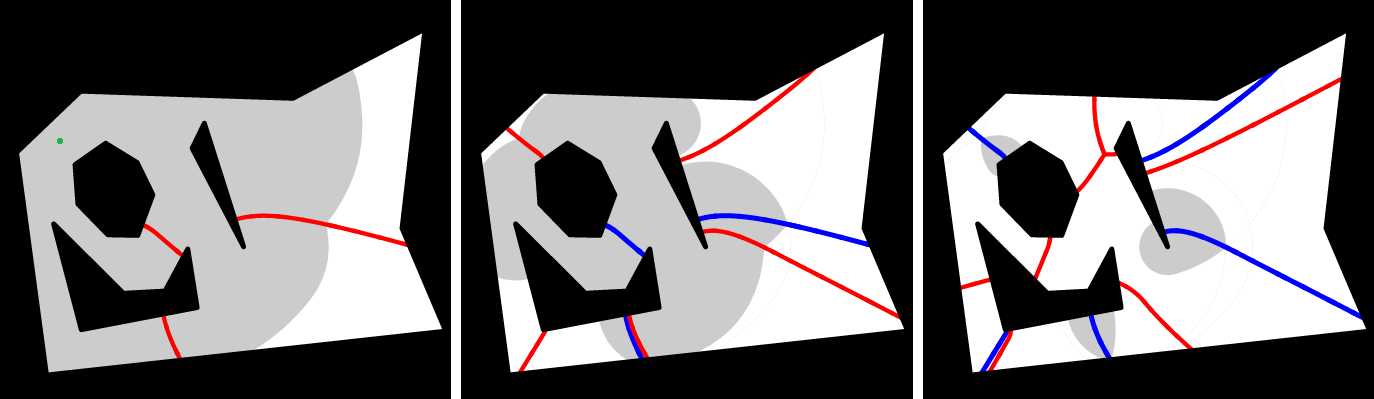k-floor as k-th homotopic shortest path map It turns out that k-walls and (k-1)-walls are comprised of the points that have two homotopically different k-paths. In other words, if we cut P along the walls into cells, then k-paths to any point within one cell will "have the same homotopy type" (we use quotes here since homotopy types are defined only for paths with the same endpoint, but the definition can be extended to compare homotopy types also for paths ending at different points; see the paper for details). In this sense the cells define the homotopic k-th shortest paths map (or k-SPM) -- a generalization of the 1-SPM from 1-paths to k-paths for arbitrary k>1. The figure below shows k-paths, for k=1,2,3, to a point: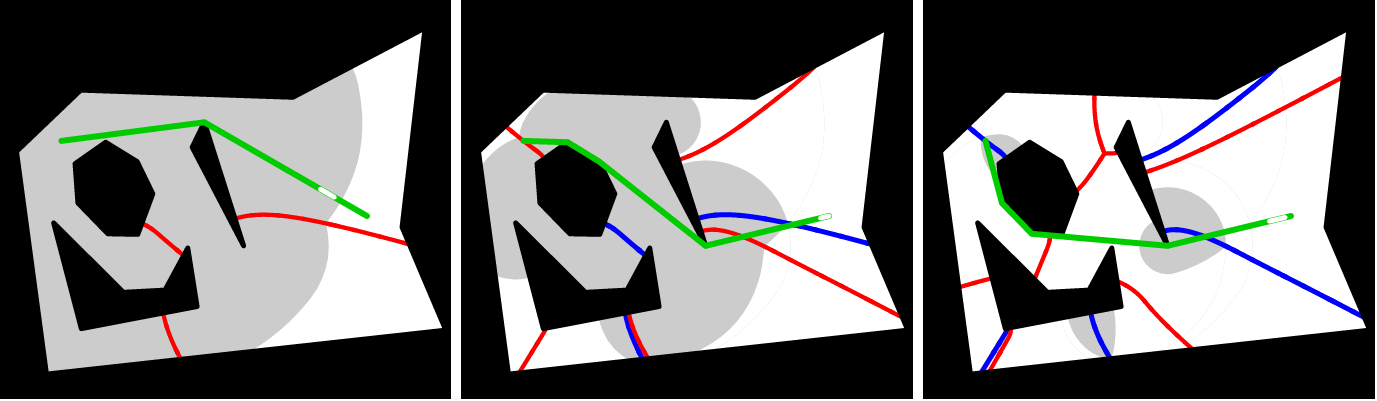The next figure shows the k-paths to a point on the other side of the 1-wall; it can be seen that 1-path and 2-path have "changed" their homotopy types: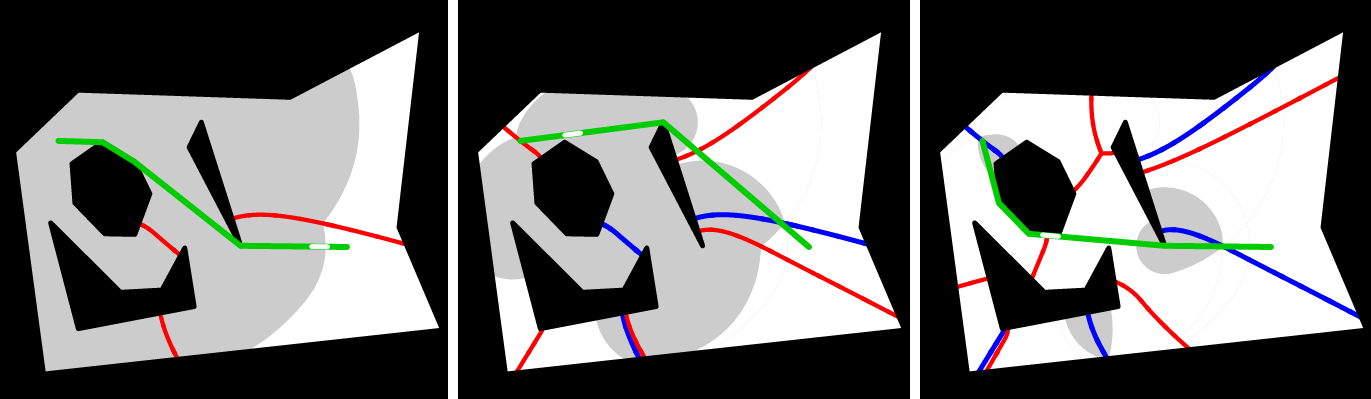The next figure shows the k-paths to a point on the other side of the 2-wall; it can be seen that 2-path and 3-path have "changed" their homotopy types: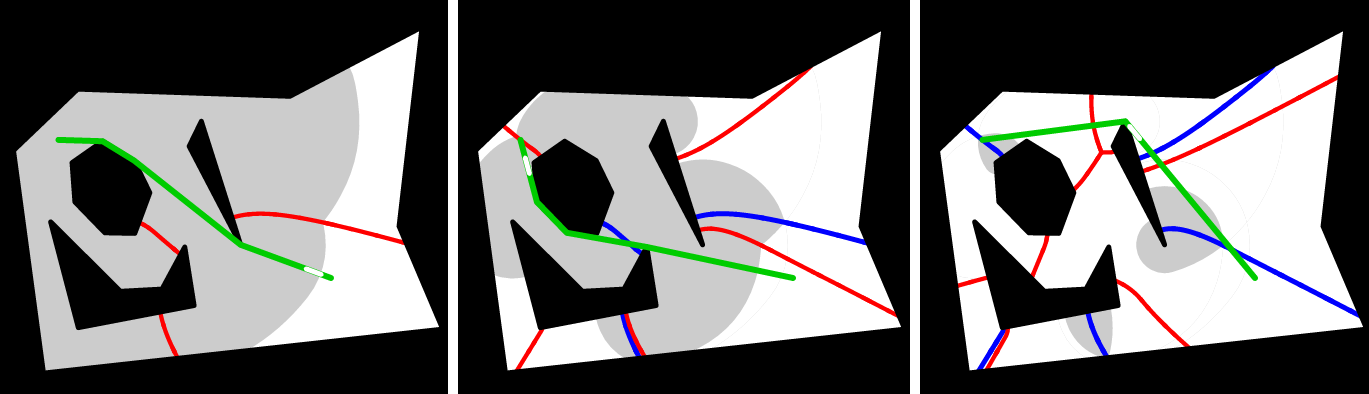k-path as 1-path in the garage By construction, no wavelet collision happens in the garage until the top floor; the wavelets collide and trace walls only at the k-floor (these walls are 1-walls for the garage and k-walls for P). Hence, the shortest path map (1-map) in the garage is obtained by simply slicing the top floor along the k-walls. The most interesting structural property of the k-paths and the garage is as follows: Let q be a point in P, let q' be the copy of q on the k-floor and let p' be the shortest path from q' to s in the garage; then the k-path to q in P is obtained by dropping down p' onto the base sheet, P. That is, the path from q to s starts on the k-floor, goes to a (k-1)-wall, uses it to get down to (k-1)-floor, then uses (k-1)-floor to reach a (k-2)-wall, uses it as a ramp down to the (k-3)-floor, and so on, until crossing a 1-wall to get on the 1-floor, on which the path goes to s. The first canvas in the figure below shows the 3-floor and a 3-path; the path crosses a 2-wall (blue) on the 3-floor. The subpath after the crossing point is a 2-path, and the next canvas shows the 2-floor and the subpath; the subpath crosses a 1-wall (blue) on the 2-floor and gets down to the 1-floor. The rest of the path (shown in the last canvas) is just the shortest path (1-path) to s.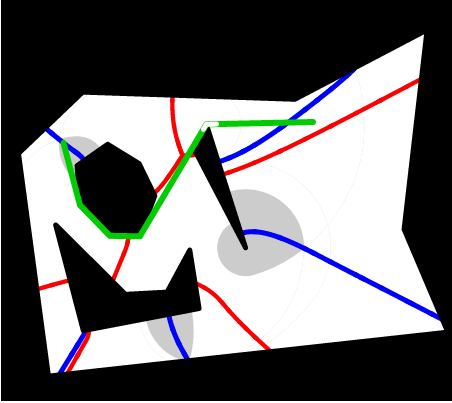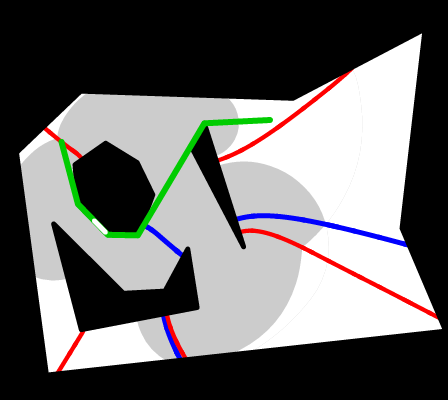The applet The applet visualizes how the wave propagates in the floors of the garage (8 floors are shown). Each canvas is a floor. The 1st canvas shows wave propagation on the 1st floor of the garage (equivalently -- wave propagation in P), the 2nd -- on the 2nd floor, etc. On a k-floor, the k-walls are red and the (k-1)-walls are blue. Hover the mouse over a point to see the k-paths to it (green); a white worm moves along the path to signify how the path loops around holes (the worm is useful if a k-path loops around a hole multiple times -- try an instance with only one small hole). Use the slider to go back and forth in the wave propagation.